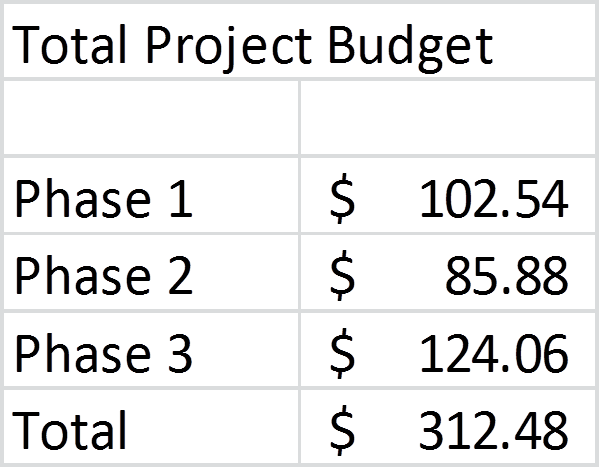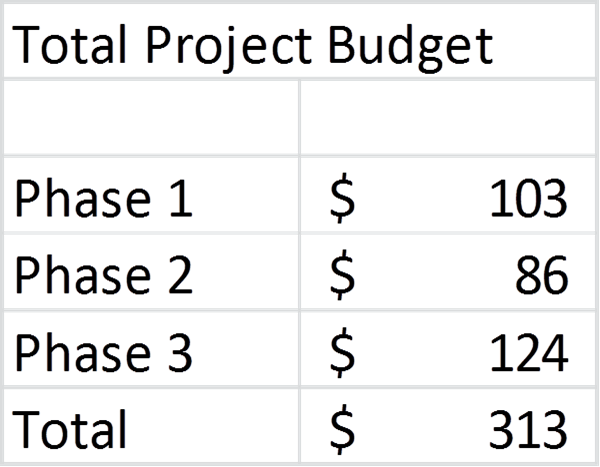# Fixing the Excel Rounding Error; Issue #329 January 20, 2015

When an executive sees a calculation error on your slide, how does it affect their view of the information you are presenting? It makes them question all the other data and analysis you did. But you checked your analysis and it is correct, so why is there an error on your slides? That’s what I want to talk about in this article.

Here is an example of what I see far too often on a slide.This is a set of summary cells copied from a much larger analysis done in Excel. The actual total of the three numbers shown is \$313, not \$312. So how did this error occur? Due to rounding in Excel. Here is what the original cells looked like before applying the format to reduce the number of decimal places:When the decimal places are shown, the math is correct. So what happens? When you apply the cell format to reduce the decimal places, Excel rounds the number in each cell individually. It does not look at whether the cell contains a formula or not. So when the numbers are rounded to zero decimal places, the Total is not adjusted to account for the rounding of each cell used in the formula. The result is that the sum is now incorrect.

How can you fix this problem in Excel before you copy the figures into PowerPoint? You need to round each of the cells to the desired decimal places before you sum the numbers in Excel. By using the ROUND() function in Excel to round each number to zero decimal places, then summing those cells, we get the following.Now the math is correct and the executives don’t have a reason to question the accuracy of the other data you are presenting (at least from a calculation perspective).

If you use Excel to create summary tables for use in your presentations, use this “rounding before adding” technique to make sure you eliminate the rounding errors that often occur in presentations to executives.